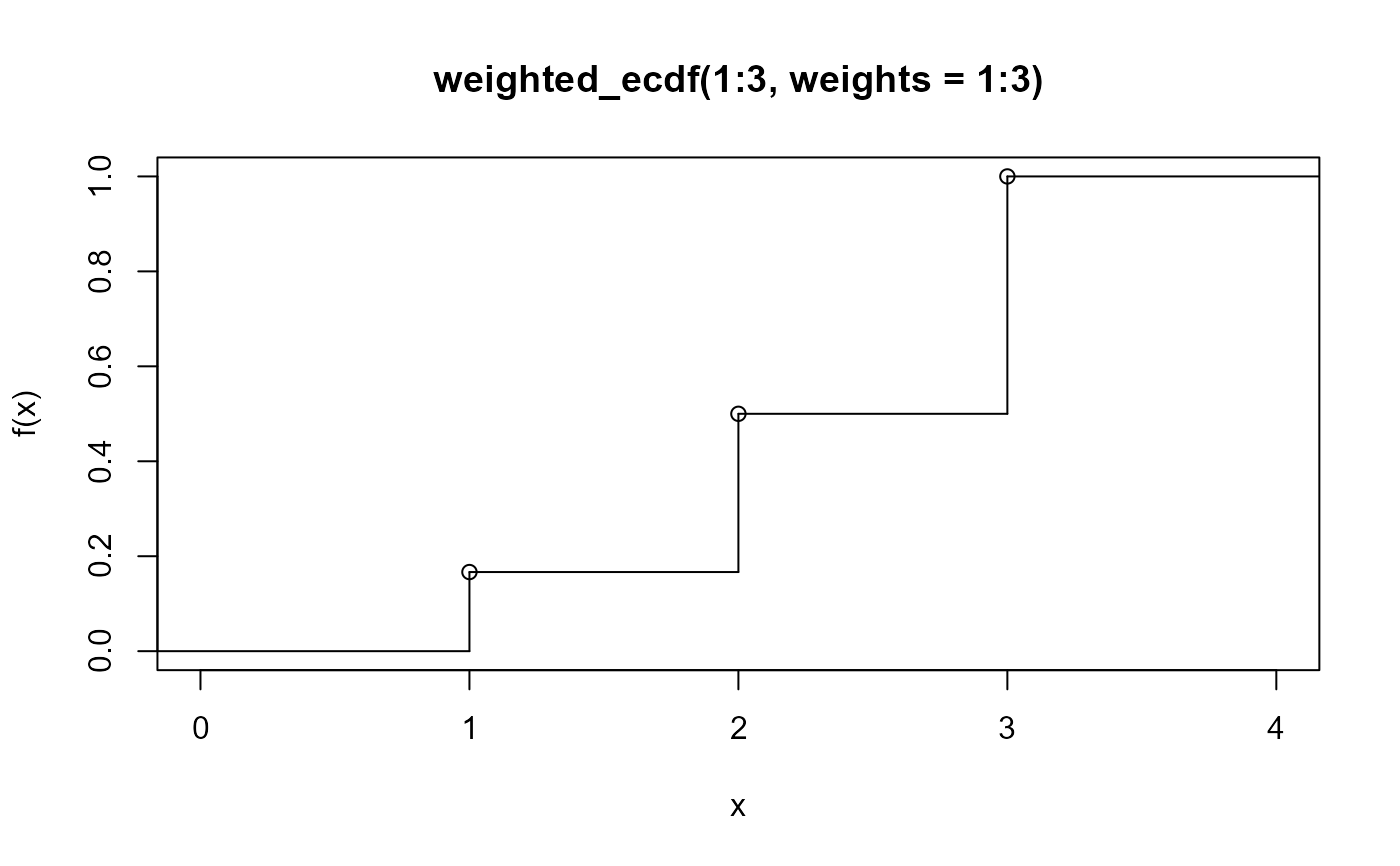A variation of ecdf() that can be applied to weighted samples.

weighted_ecdf(x, weights = NULL, na.rm = FALSE)

## Arguments

x

numeric vector: sample values

weights

Weights for the sample. One of:

• numeric vector of same length as x: weights for corresponding values in x, which will be normalized to sum to 1.

• NULL: indicates no weights are provided, so the unweighted empirical cumulative distribution function (equivalent to ecdf()) is returned.

na.rm

logical: if TRUE, corresponding entries in x and weights are removed if either is NA.

## Value

weighted_ecdf() returns a function of class "weighted_ecdf", which also inherits from the stepfun() class. Thus, it also has plot() and print()

methods. Like ecdf(), weighted_ecdf() also provides a quantile() method, which dispatches to weighted_quantile().

## Details

Generates a weighted empirical cumulative distribution function, $$F(x)$$. Given $$x$$, a sorted vector (derived from x), and $$w_i$$, the corresponding weight for $$x_i$$, $$F(x)$$ is a step function with steps at each $$x_i$$ with $$F(x_i)$$ equal to the sum of all weights up to and including $$w_i$$.

weighted_quantile()

## Examples

weighted_ecdf(1:3, weights = 1:3)
#> Step function
#> Call: weighted_ecdf(1:3, weights = 1:3)
#>  x[1:3] =      1,      2,      3
#> 4 plateau levels =      0, 0.16667,    0.5,      1
plot(weighted_ecdf(1:3, weights = 1:3))quantile(weighted_ecdf(1:3, weights = 1:3), 0.4)
#>  2.2